# How to select random sample in Excel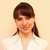by , updated on

This tutorial will teach you a few quick ways to randomly select names, numbers or any other data. You will also learn how to get a random sample without duplicates and how to randomly select a specified number or percentage of cells, rows or columns in a mouse click.

Whether you do market research for a new product launch or evaluating the results of your marketing campaign, it is important that you use an unbiased sample of data for your analysis. And the easiest way to achieve this is to get random selection in Excel.

## What is random sample?

Before discussing sampling techniques, let's provide a bit of background information about random selection and when you might want to use it.

In probability theory and statistics, a random sample is a subset of data selected from a larger data set, aka population. Each element of a random sample is chosen entirely by chance and has an equal probability of being selected. Why would you need one? Basically, to get a non-biased representation of the total population.

For example, you want to conduct a little survey among your customers. Obviously, it would be unwise to send out a questionnaire to each single person in your multi-thousand database. So, whom do your survey? Will that be 100 newest customers, or the first 100 customers listed alphabetically, or 100 people with the shortest names? None of these approaches fit your needs because they are innately biased. To get an impartial sample where everyone carries an equal opportunity of being chosen, do a random selection by using one of the methods described below.

## Excel random selection with formulas

There's no built-in function to randomly pick cells in Excel, but you can use one of the functions to generate random numbers as a workaround. These probably cannot be called simple intuitive formulas, but they do work.

### How to select a random value from a list

Supposing you have a list of names in cells A2:A10 and you want to randomly select one name from the list. This can be done by using one of the following formulas:

`=INDEX(\$A\$2:\$A\$10,RANDBETWEEN(1,COUNTA(\$A\$2:\$A\$10)),1)`

or

`=INDEX(\$A\$2:\$A\$10,RANDBETWEEN(1,ROWS(\$A\$2:\$A\$10)),1)`

That's it! Your random name picker for Excel is all set up and ready to serve: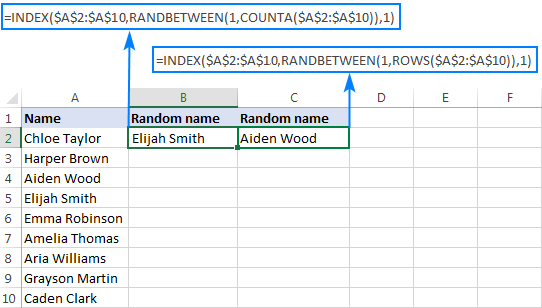Note. Please be aware that RANDBETWEEN is a volatile function, meaning it will recalculate with every change you make to the worksheet. As the result, your random selection will also change. To prevent this from happening, you can copy the extracted name and paste it as value to another cell (Paste Special > Values). For the detailed instructions, please see How to replace formulas with values.

Naturally, these formulas can not only pick random names, but also select random numbers, dates, or any other random cells.

#### How these formulas work

In a nutshell, you use the INDEX function to extract a value from the list based on a random row number returned by RANDBETWEEN.

More specifically, the RANDBETWEEN function generates a random integer between the two values you specify. For the lower value, you supply the number 1. For the upper value, you use either COUNTA or ROWS to get the total row count. As the result, RANDBETWEEN returns a random number between 1 and the total count of rows in your dataset. This number goes to the row_num argument of the INDEX function telling it which row to pick. For the column_num argument, we use 1 since we want to extract a value from the first column.

Note. This method works well for selecting one random cell from a list. If your sample is supposed to include several cells, the above formula may return several occurrences of the same value because the RANDBETWEEN function is not duplicate-free. It is especially the case when you are picking a relatively big sample from a relatively small list. The next example shows how to do random selection in Excel without duplicates.

### How to randomly select in Excel without duplicates

There are a few ways to select random data without duplicates in Excel. Generally, you'd use the RAND function to assign a random number to each cell, and then you pick a few cells by using an Index Rank formula.

With the list of names in cells A2:A16, please follow these steps to extract a few random names:

1. Enter the Rand formula in B2, and copy it down the column:
`=RAND()`
2. Put the below formula in C2 to extract a random value from column A:

`=INDEX(\$A\$2:\$A\$16, RANK(B2,\$B\$2:\$B\$16), 1)`

3. Copy the above formula to as many cells as many random values you want to pick. In our case, we copy the formula to four more cells (C2:C6).

That's it! Five random names are extracted without duplicates: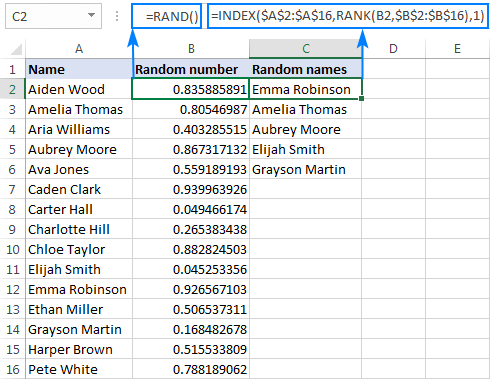#### How this formula works

Like in the previous example, you use the INDEX function to extract a value from column A based on a random row coordinate. In this case, it takes two different functions to get it:

• The RAND formula populates column B with random numbers.
• The RANK function returns the rank a random number in the same row. For example, RANK(B2,\$B\$2:\$B\$16) in cell C2 gets the rank of the number in B2. When copied to C3, the relative reference B2 changes to B3 and returns the rank of the number in B3, and so on.
• The number returned by RANK is fed to the row_num argument of the INDEX function, so it picks the value from that row. In the column_num argument, you supply 1 because you want to extract a value from the first column.

A word of caution! As shown in the screenshot above, our Excel random selection contains only unique values. But theoretically, there is a slim chance of duplicates appearing in your sample. Here's why: on a very large dataset, RAND might generate duplicate random numbers, and RANK will return the same rank for those numbers. Personally, I've never got any duplicates during my tests, but in theory, such probability does exist.

If you are looking for a bulletproof formula to get a random selection with only unique values, then use RANK + COUNTIF or RANK.EQ + COUNTIF combination instead of just RANK. For the detailed explanation for the logic, please see Unique ranking in Excel.

The complete formula is a bit cumbersome, but 100% duplicate-free:

`=INDEX(\$A\$2:\$A\$16, RANK.EQ(B2, \$B\$2:\$B\$16) + COUNTIF(\$B\$2:B2, B2) - 1, 1)`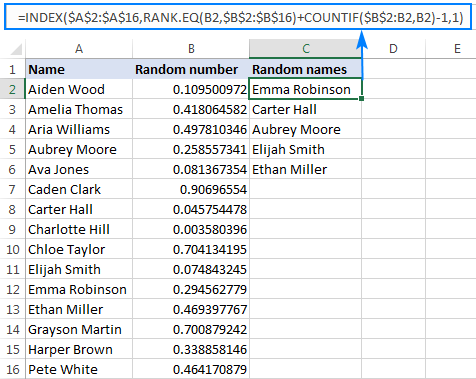Notes:

• Like RANDBETWEEN, the Excel RAND function also generates new random numbers with each recalculation of your worksheet, causing the random selection to change. To keep your sample unchanged, copy it and paste somewhere else as values (Paste Special > Values).
• If the same name (number, date, or any other value) appears more than once in your original data set, a random sample might also contain several occurrences of the same value.

More ways to get a random selection with no repeats in Excel 365 - 2010 are described here: How to get random sample in Excel without duplicates.

### How to select random rows in Excel

In case your worksheet contains more than one column of data, you can select a random sample in this way: assign a random number to each row, sort those numbers, and select the required number of rows. The detailed steps follow below.

1. Insert a new column to the right or to the left of your table (column D in this example).
2. In the first cell of the inserted column, excluding the column headers, enter the RAND formula: `=RAND()`
3. Double-click the fill handle to copy the formula down the column. As the result, you will have a random number assigned to each row.
4. Sort the random numbers largest to smallest (sorting in ascending order would move the column headers at the bottom of the table, so be sure to sort descending). For this, head over to the Data tab > Sort & Filter group, and click the ZA button. Excel will automatically expand the selection and sort the entire rows in random order.

If you are not quite satisfied with how your table has been randomized, hit the sort button again to resort it. For the detailed instructions, please see How to randomly sort in Excel.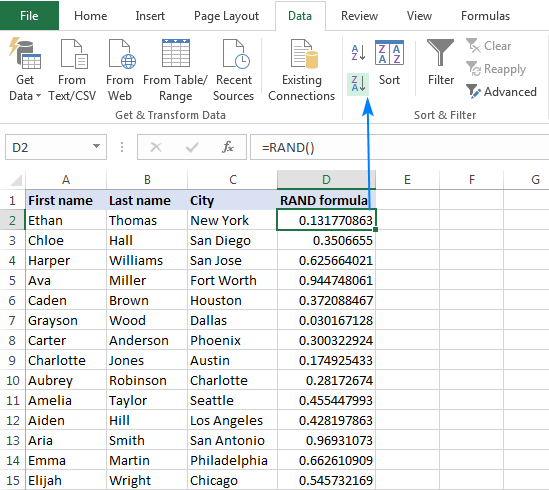5. Finally, select the required number of rows for your sample, copy them and paste to wherever you like.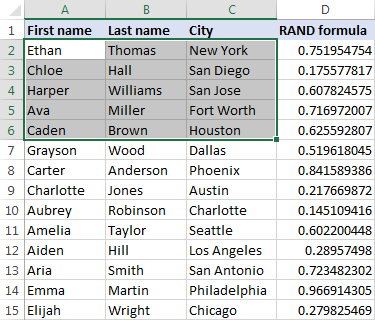To have a closer look at the formulas discussed in this tutorial, you are welcome to download our sample workbook to Excel Random Selection.

## How to randomly select in Excel with Randomize tool

Now that you know a handful of formulas to get a random sample in Excel, let's see how you can achieve the same result in a mouse click.

With Random Generator for Excel included in our Ultimate Suite, here's what you do:

1. Select any cell in your table.
2. Go to the Ablebits Tools tab > Utilities group, and click Randomize > Select Randomly:3. On the add-in's pane, choose what to select: random rows, random columns or random cells.
4. Specify the number or percentage for the desired sample size.
5. Click the Select button. Done!

For example, this is how we can select 5 random rows from our sample data set: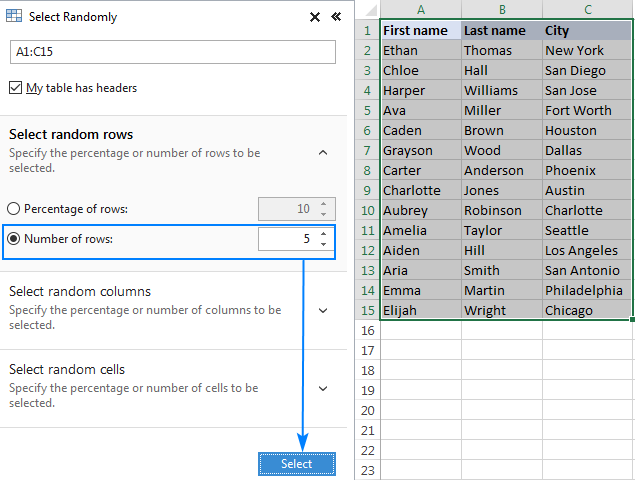And you will get a random selection in a second: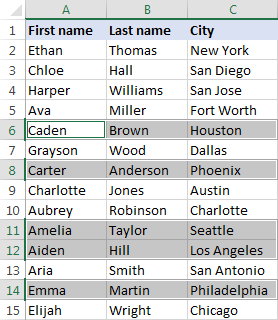Now, you can press Ctrl + C to copy your random sample, and then press Ctrl + V to paste it to location in the same or another sheet.

If you'd like to test the Randomize tool in your worksheets, just grab a trial version of Ultimate Suite below. In case your are using Google spreadsheets, you may find our Random Generator for Google Sheets useful.